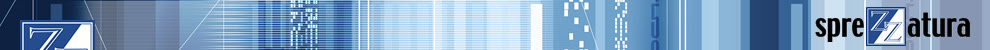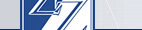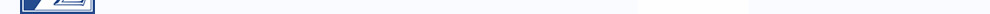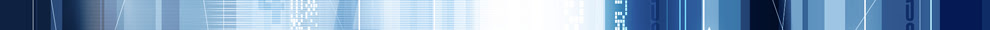By apk | Tuesday, 4 December 2012 11:42 | 0 Comments
The equals operator, or "=" to represent it symbolically. A simple programming standard; something you learned in the first 10-15 minutes of programming. Even with the simple statement

= 1

which we all read out as

x equals 1

we know that's not really completely true. It's more accurately

Now, x should be set to 1

or even more accurately,

assign 1 to the variable x

Therefore, you could be safe in assuming that "=" isn't actually the equals operator at all, it's actually the assign operator. History buffs will recall that the original BASIC syntax was actually

LET X = 1

a construct allowed in ARev up through 3.12. But, simple assignment statements aren't all that the "=" operator is used for. In the statement

if x = 1 then gosub here else gosub there

x is not assigned the value of 1 when the statement is executed. Here, "=" is used a comparison operator, like ">" or "<". With that knowledge, is there anyway we can use "=" as a comparison operator outside of an "if" statement, and if so, why would we want to do that? As you've probably surmised, the answer to that -question is "yes", otherwise, what would be the point of this article? Consider the following:

= y = z

In some languages, that will assign the value of "z" to "y" and then to "x". However, Basic+ does not work this way. As we alluded to many years ago in SENL Volume 2, Issue 1 (warning:PDF link), Basic+ is an expression based language. What happens in the above sentence is that "x" is assigned the value of the expression on the right side of the first "=", which is "y = z". This still leaves us with the question of, what is "y = z" in this context? To answer that, we need to go back to our "if...then" statement. An "if...then" statement is, in its purest form,

if TRUE\$ then something else somethingElse

This means for statements like

if x = 1 then gosub here else gosub there

to work, then the "x = 1" must evaluate to true. This means that in "x = y = z", the "y = z" portion is evaluated as a comparison operator like it does in an "if...then" statement. "y = z" will evaluate to a logical TRUE\$ if "y" equals "z", and to a logical FALSE\$ if not. Therefore the following two lines of code are functionally equivalent:

if y = z then x = TRUE\$ else x = FALSE\$

= y = z

As to which one is clearer, that's programmatic style. I find this useful in a variety of settings, but mostly in setting flags.

* // overFlag is set to TRUE\$ if invVal is greater than 10,000
* // or if there is a value in the pastDueAmt
overFlag = ( ( invVal >= 10000) or ( pastDueAmt > 0 ) )

* // the prompt is enabled if the record is not readOnly
* // and the user has been defined as a super user
enabled = ( ( not(readOnly)) and (superUser = TRUE\$ ) )

If you notice, I'm overly generous with my use of parentheses/brackets in these statements. Part of that is personal style, I like putting my statements inside parentheses/brackets. However, these statements can be tricky to read, and operator precedence starts to come into play. It's best to put things in parentheses/brackets to ensure what you want to happen happens when you want it to happen.

The reason we decided to post this, was based on some code we needed to fix for a client. In there we found a large section of lines similar to

disArray := @VM : len( @record<43> ) = 0

This was in a large block of code setting ENABLED states in a window. It wasn't working. What's happening here is that the system takes "@VM:len( @record<43>)", compares that to 0, and then appends it to disArray because the : between the @VM and the len statement executes higher in the precedence order than the len statement with the " =0" comparison. This resulted in "disArray" being filled with what appeared to be a large binary string. I just want to mention here that that's the actual variable name, and I couldn't help but laugh at the irony.

To help clarify, here's a quick debugger view of a sample test program, and its results.In the variable "correct", you can see that in its initial concatenation value, we have "value²0". This is because the system takes the contents of correct ("value"), concatenates an @VM, then concatenates the result of the "len(myVar) = 0" comparison, which is a logical FALSE\$.

In the variable "incorrect", you can see that in its initial concatenation value, we have "value0". This is because the system first evaluates the expression "@VM:len(myVar)" which results in "²3". It uses the "=" operator to compare that to 0. This results in a logical FALSE\$. This is then concatenated onto "incorrect", giving us a variable containing "value0".

The next two lines create copies of our variable so we can examine them in stages.

The variable "correct2" is assigned its value the same was as "correct" was. The system takes the contents of "correct2" ("value²0"), concatenates an @VM, then concatenates the result of the "len(myVar) = 3" comparison, which is a logical TRUE\$. This results in "value²0²1".

The variable "incorrect2" is assigned its value the same way as "incorrect" was. This is because the system first evaluates the expression "@VM:len(myVar)" which results in "²3". It uses the "=" operator to compare that to 3. This results in a logical FALSE\$. This is then concatenated onto "incorrect", giving us a variable containing "value00".

There is an extended operator which can be used to help clarify your code. Basic+ supports the "==" operator when "equals" is used a comparison operator. For example, we can modify our sample code above to be

subroutine EqualsExamples( void )

myVar = "abc"

correct = "value"
incorrect = "value"

correct := @VM : (len( myVar) == 0)
incorrect := @VM : len( myVar) == 0

correct2 = correct
incorrect2 = incorrect

correct2 := @VM : (len( myVar) == 3 )
incorrect2 := @VM : len( myVar) == 3

debug

return

The use of the "==" operator helps differentiate when "=" is being used as an assignment and when it is being used a comparison. The "==" operator also works in "if...then" statements.

There is also an additional related syntax which is little known or used. Consider the following:

color = if ( amount > MAX_AMOUNT\$ ) then RED\$ else GREEN\$

This will assign RED\$ to color if amount is greater than MAX_AMOUNT, otherwise color will be set to GREEN\$.

This "if...then" construct must be on a single line and the TRUE\$ and FALSE\$ values must be a single expression value.

An expression value, as mentioned above, is anything that can be on the right side of an assignment "=" statement. We could rework this statement so it looks something like

declare function getAppColors
....
color = if ( amount > MAX_AMOUNT\$ ) |
then getAppColors( MAX_EXCEED_COLOR\$) |
else getAppColors( NORMAL_COLOR\$ )

This construct for assigning a variable can help eliminate unassigned variables, since color is guaranteed to be assigned to something. We hope this explains some of the ways Basic+ evaluates various portions of the code and the use of expressions in Basic+.

As a corollary, the concepts in the article might explain why sometimes runtime errors occur in places you don't expect, for example, an unassigned return value in a function will break in the calling program, and not in the actual function. The short reason for this is that the "return opcode" just returns what's on the stack, so it successfully returns an unassigned.

Consider these short function and subroutine snippets.

...
results = param1 * something
return result

~~~~~~~~~~~~~~~~~~~~~~~~~~~~~~~~~~~~~~~~~~~~~~~

subroutine myProg( void )
...
....
return

When "result" is returned from badFunc, an unassigned memory area is returned. This means the expression "badFunc( someAssignedVar)" is what's unassigned, so Basic+ will attempt to assign an unassigned variable to myVar, meaning myProg will break on line X. This is why when you have invalid handles in "SELECT..BY" statements or in "XLATE" clauses, RTP12 and RTP16 don't always break, but the calling program sometimes does.

The little "=" operator. Who'd have thought it was so complicated?

Labels:Making Databases Happen UK +44 (0) 20 8912 1010 USA +1 267 238 3814